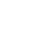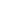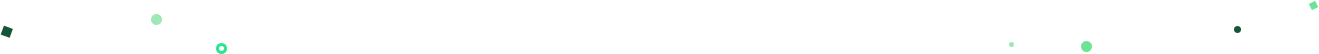Introducing Visual Explorer, a new tool for data visualization.Learn more## Mode Studio

The Collaborative Data Science Platform

# SQL SUM

Starting here? This lesson is part of a full-length tutorial in using SQL for Data Analysis. Check out the beginning.

In this lesson we'll cover:

## The SQL SUM function

`SUM` is a SQL aggregate function. that totals the values in a given column. Unlike `COUNT`, you can only use `SUM` on columns containing numerical values.

The query below selects the sum of the `volume` column from the Apple stock prices dataset:

``````SELECT SUM(volume)
FROM tutorial.aapl_historical_stock_price
``````

An important thing to remember: aggregators only aggregate vertically. If you want to perform a calculation across rows, you would do this with simple arithmetic.

You don't need to worry as much about the presence of nulls with `SUM` as you would with `COUNT`, as `SUM` treats nulls as 0.

## Practice Problem

Write a query to calculate the average opening price (hint: you will need to use both `COUNT` and `SUM`, as well as some simple arithmetic.).

Try it out See the answer

Next Lesson

SQL MIN/MAX# Get our weekly data newsletter

Work-related distractions for every data enthusiast.